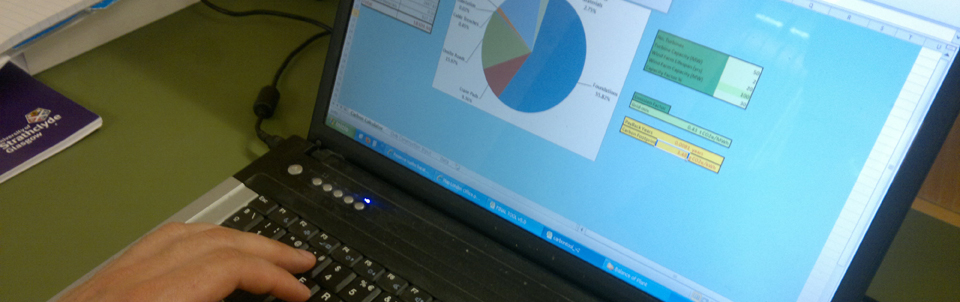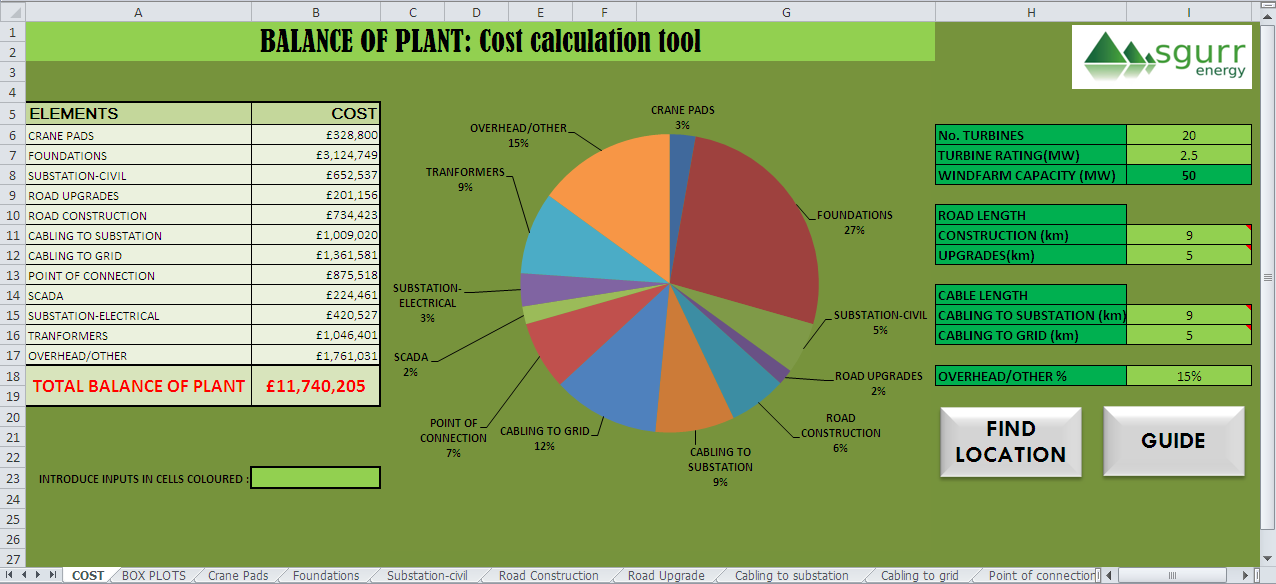## Instructions

Each tool has input paramaters for the user to input and this will give a figure relating to the output desired.

The tabbed menus are for data input, this can be from previous projects, tenders and other resources. This data is used to perform the calculations.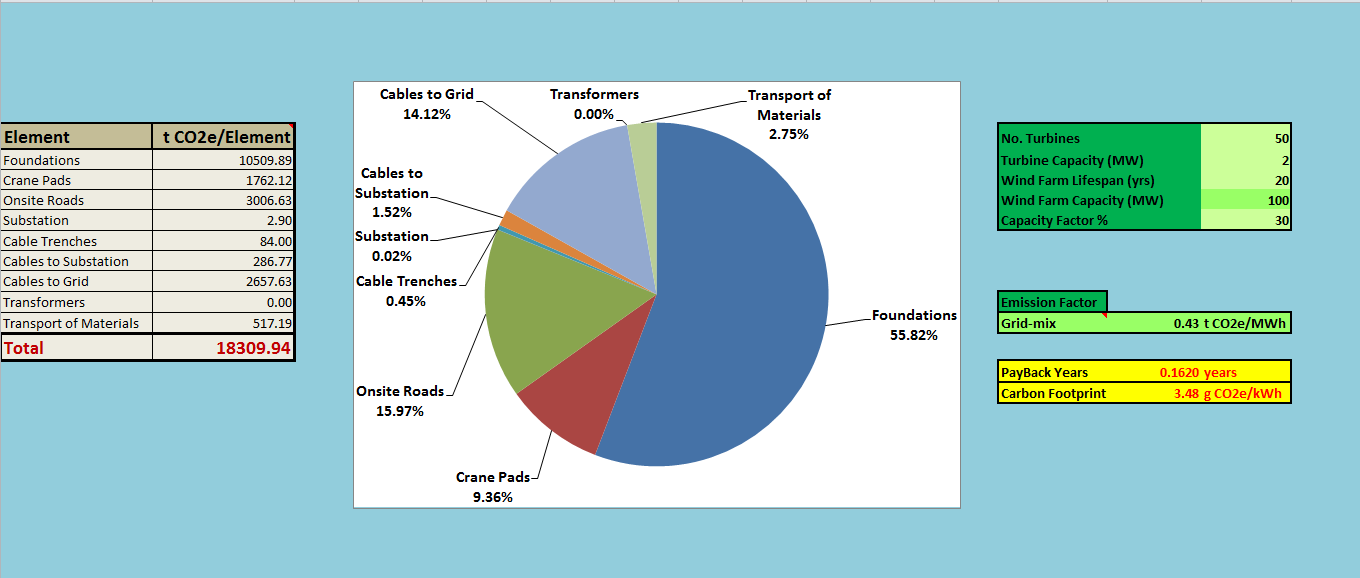## Instructions

### Cost Tool

The tool has a first sheet, labelled as ‘COST’ (Figure 1), where the site characteristics are entered in the green boxes to the right and the results are shown numerically in the table to the left and in the central Pie chart.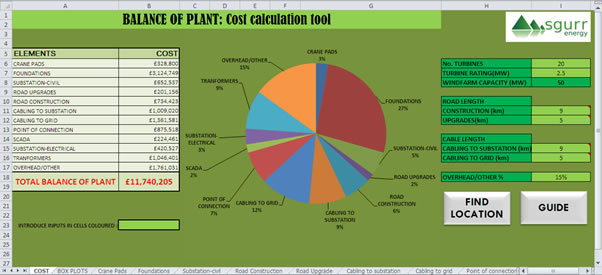Figure 1

On the second sheet, labelled as ‘BOX PLOTS’(Figure 2), the cost for the different categories is shown using box plots. Also numerical data of the statistical quartiles is found here.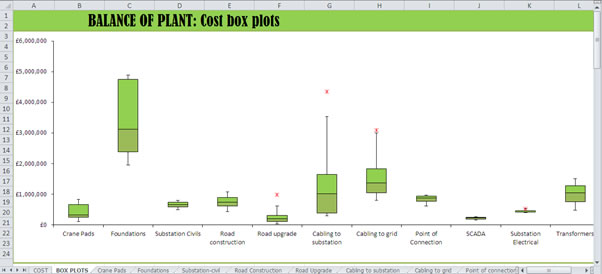Figure 2

The other 11 sheets are used as the historical data base (Figure 3). Each sheet contains the cost data attributed to the category named on that sheet. The user input of information from existing projects is entered in the white blank columns to the left while the others show results of the calculations. Average costs and a graph relating the costs with turbine size are found to the right. The sizing factor is entered in the blue box to the left, check ‘Methodology’ section to find out more about this sizing factor.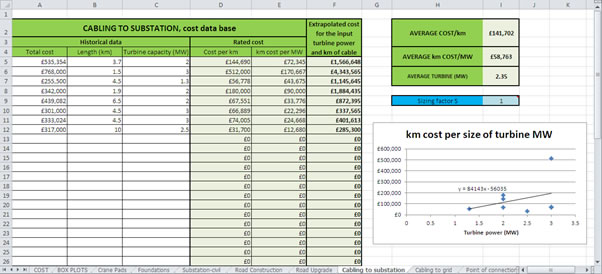Figure 3

### Carbon tool

The first page is entitled Carbon Calculator and the inputs are inserted in the light green boxes on the right; emissions factor can be selected; the Carbon Payback and Intensity are in the yellow boxes.Figure 4

The second page is entitled Civils Construction Inputs and values are entered in the white boxes and the outputs for each elements embodied carbon and transported carbon are found in the turquoise and yellow boxes circled in the figure 5.Figure 5